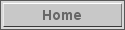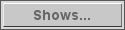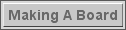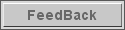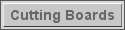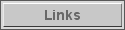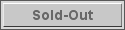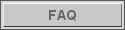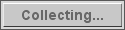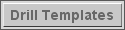www.EnumeroCribbageBoards.com
 19 in Prime Time - Series IAside from not being able to attain 19 pointsin a regulation Cribbage Hand, the number 19shows many distinct properties in the world of Prime Numbers.A sampling of these unique properties inspired this series.Source: Prime CuriosThe Dictionary of Prime Number TriviaIssued:<< chenchen tournie boards >>
Board Name
Click on Thumbnail
Purchase

19 Prime #1

Take nine pairs of numbers
(a,b) where a+b=19. Take the
sum a*b+19 for all of these
nine pairs; ALL of the sums
are primes. 19 is the
LARGEST prime that will do
this.

( \$42 )19 Prime #2

Pandigital palindromic primes
start as 19-digit numbers

( \$42 )19 Prime #3

(11, 13, 17, 19) is the first
prime quadruple (p, q, r, s)
such that
s divides pqr+1.

( \$42 )19 Prime #4

There are exactly 19 primes
between
2^5 -1 and 2^7-1.

( \$42 )19 Prime #5

The last 19 digits of
17^18 are prime.

( \$42 )19 Prime #6

The only known prime of the
form p*prime(p)^p + 1, where
p is prime.

( \$42 )19 Prime #7

19 is the smallest prime which
is the sum of three
discrete composites (4 + 6 + 9).

( \$42 )19 Prime #8

19 is the only known prime of
the
form n^n - 8. The next prime
in
this form (if it exists) will
have more than 34000 digits.

( \$42 )19 Prime #9

Both numbers reversal (19! - 1)
and reversal ( 19! + 1) are
primes.
19 is the largest known prime
with this property.

( \$42 )19 Prime #10

The largest prime that is
palindromic in Roman
numerals alphabetically is
XIX (19).

( \$42 )19 Prime #11

The first prime p such that
p^2 is the reversal of a prime
(163).

( \$42 )19 Prime #12

The following are primes: 19,
109, 1009, 10009. No other
digit can replace the 9 and
yield four primes.

( \$42 )19 Prime #13

19 is the only known number
for which both (10^n-1)/9 and
(10^n+1)/11 are primes.

( \$42 )19 Prime #14

19 is the only prime p less than
30 that does not divide 30,
where p + 30 is composite.

( \$42 )19 Prime #15

19 is the smallest prime with a
digital root of 1.

( \$42 )19 Prime #16

19 is the smallest prime of the
form 3p + 2q, where p and q
are twin primes.

( \$42 )19 Prime #17

2^19 - 19 is prime.

( \$42 )19 Prime #18

19 is the smallest prime whose
reversal is composite.

( \$42 )19 Prime #19 (o)

(19 - 1) divides (19^19 - 1)
a prime number of times.

( \$51 )